# NCERT Exemplar Class 12 Chemistry Solutions for Chapter 15 - Polymers

NCERT Class 12 Chemistry Exemplar for Chapter 15 Polymers provide you polymer questions multiple choice, polymer MCQ for NEET, Fill in the blanks, Match the following, True or False, Worksheets, polymers Class 12 important questions, exercises and assignments. Studying NCERT Class 12 Chemistry exemplar for Chapter 15 Polymers will help you learn the concept comprehensively from the exam point of view. These solutions help your preparation for the graduate entrance exam and make you well equipped to face the exam confidently.

## Download PDF of NCERT Exemplar For Class 12 Chemistry Chapter 15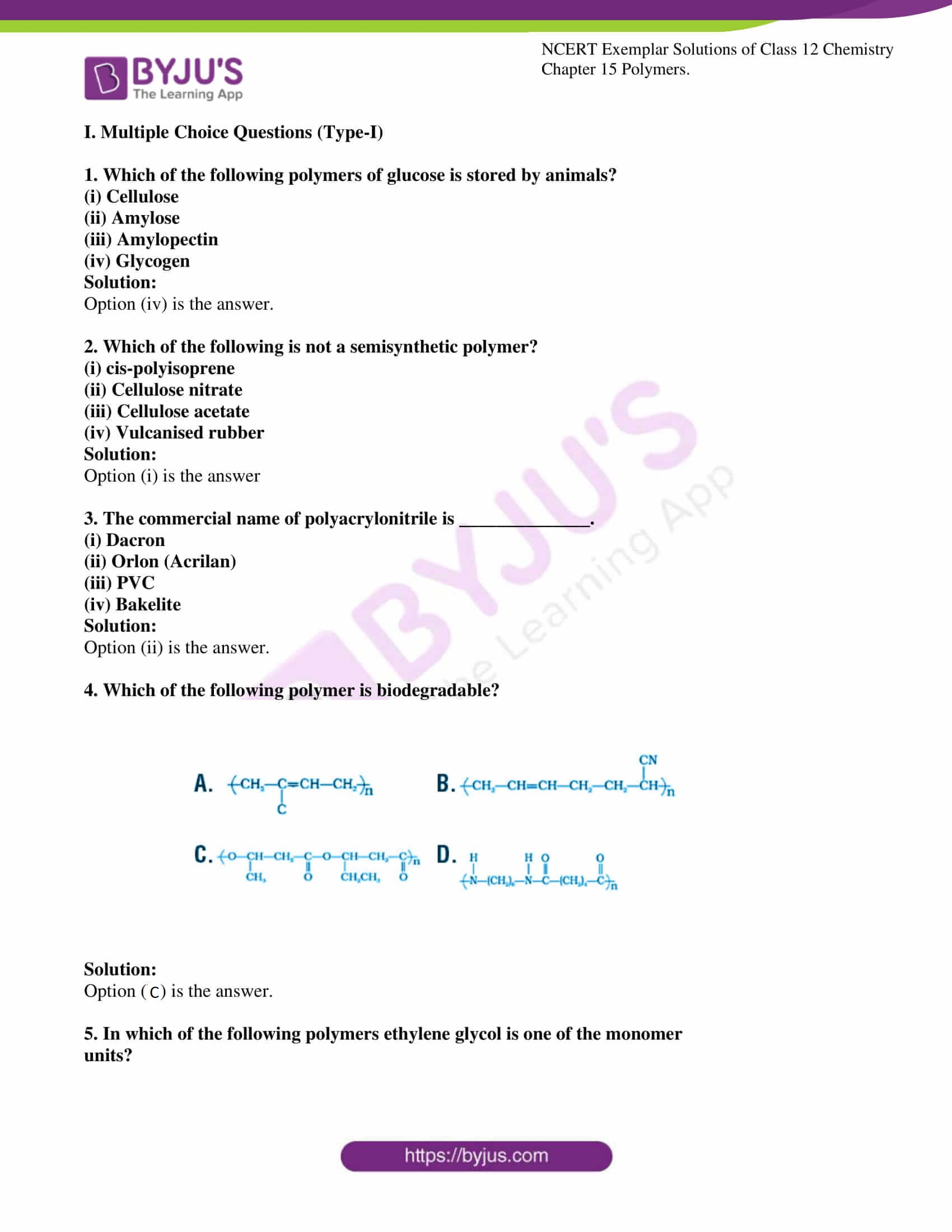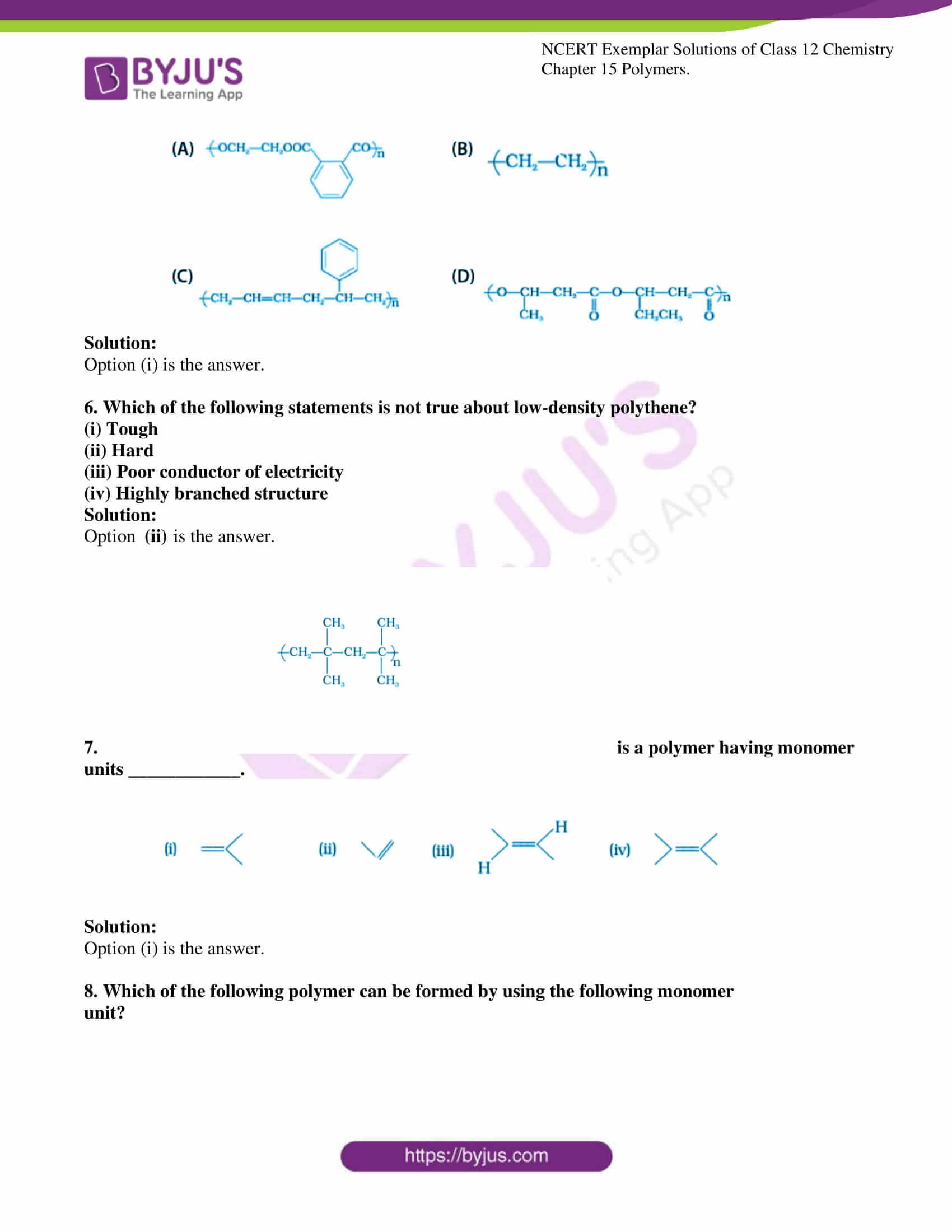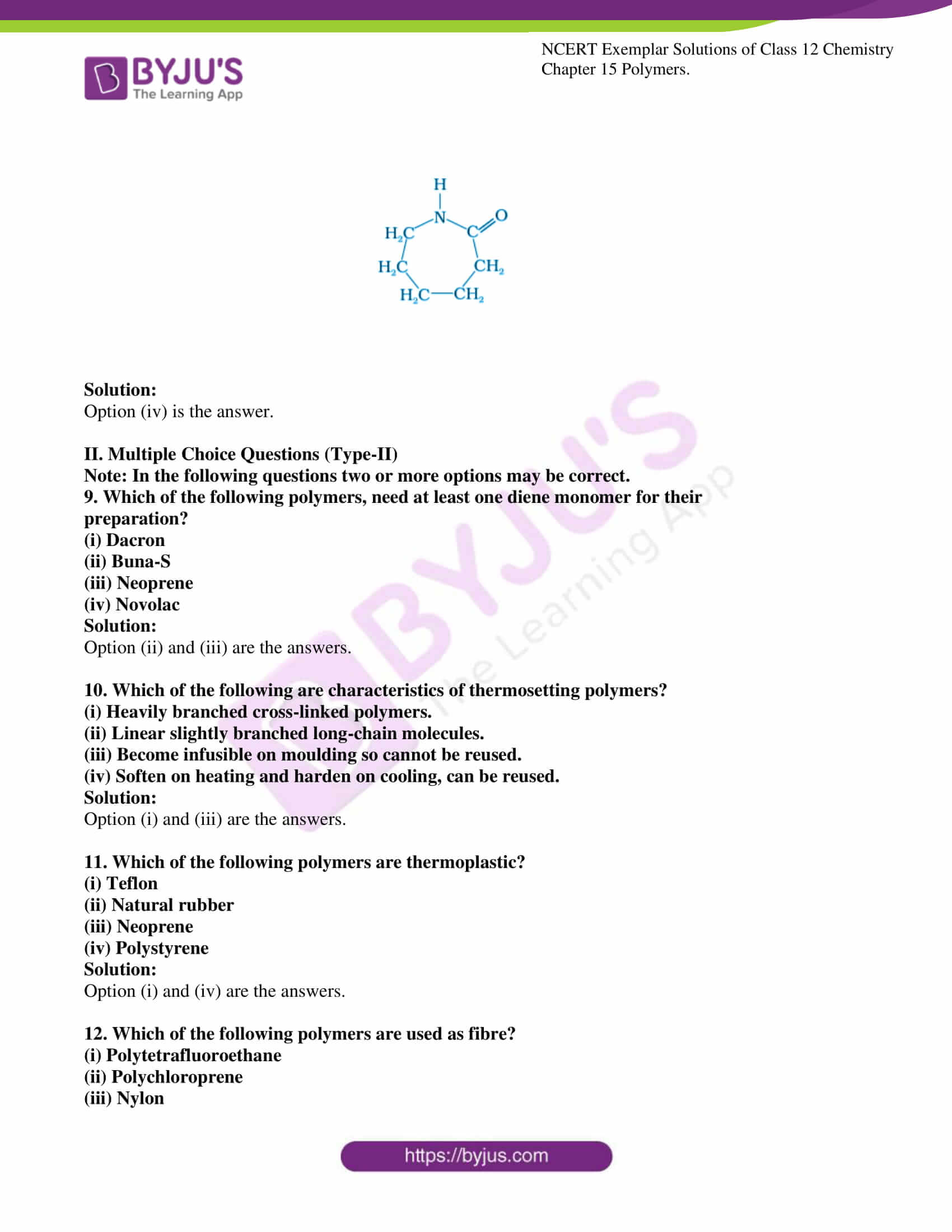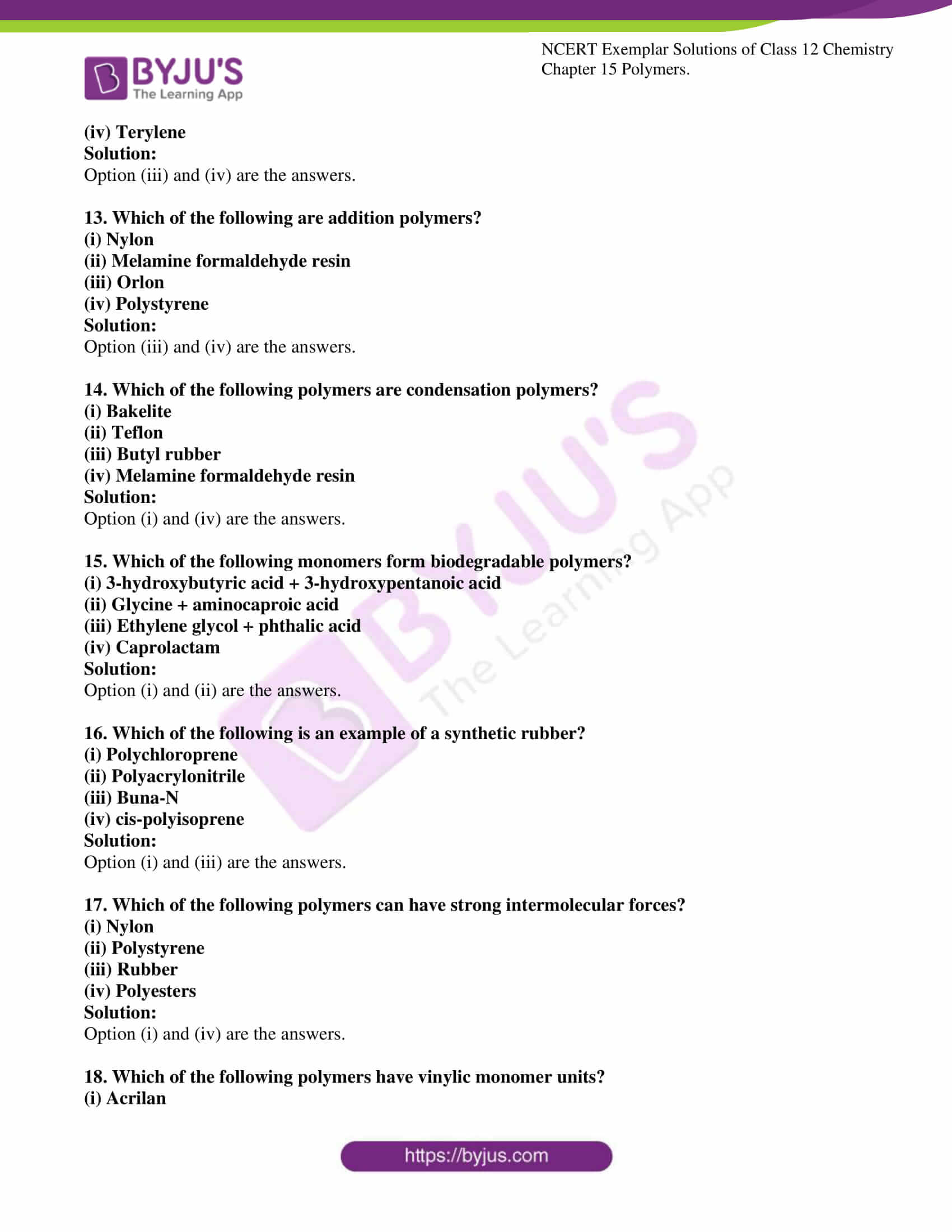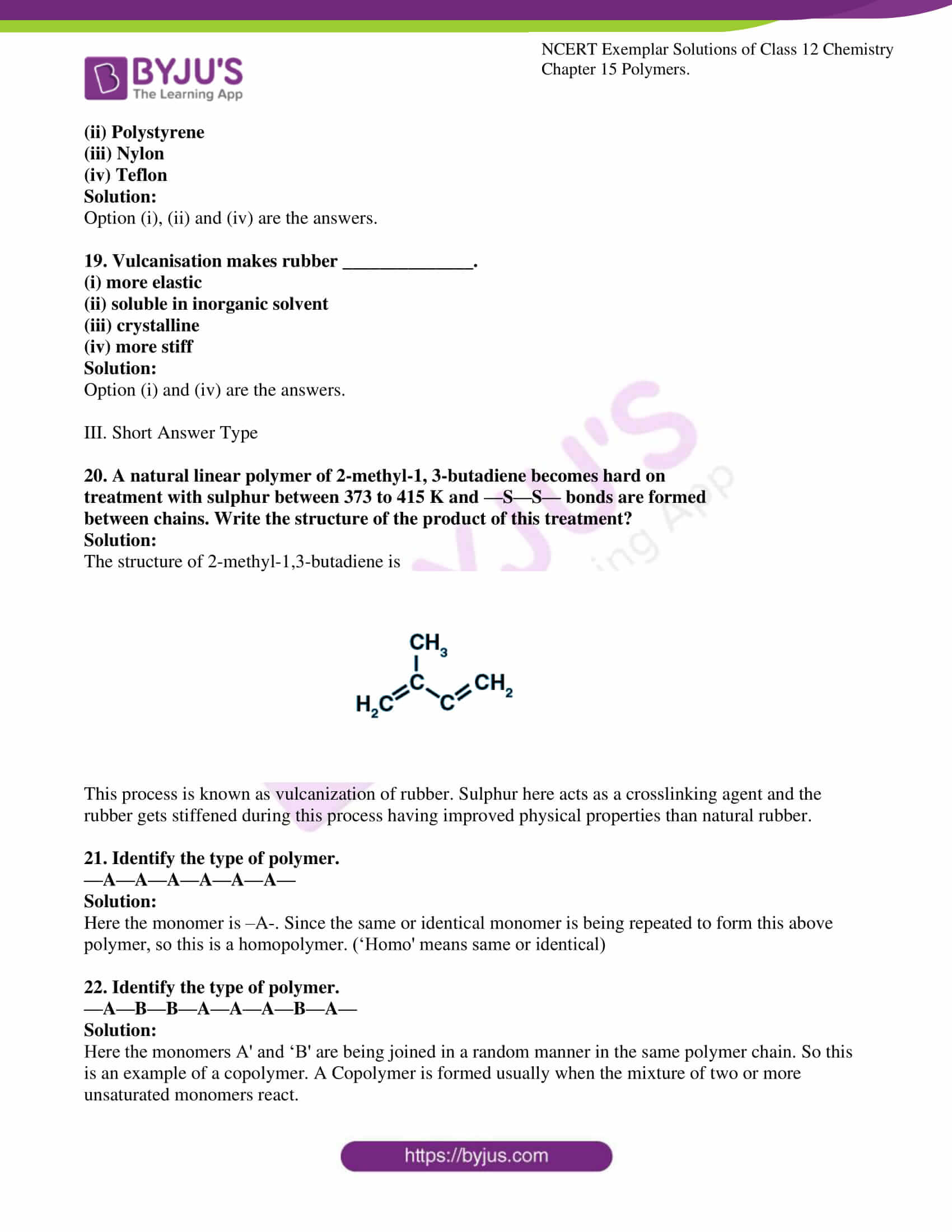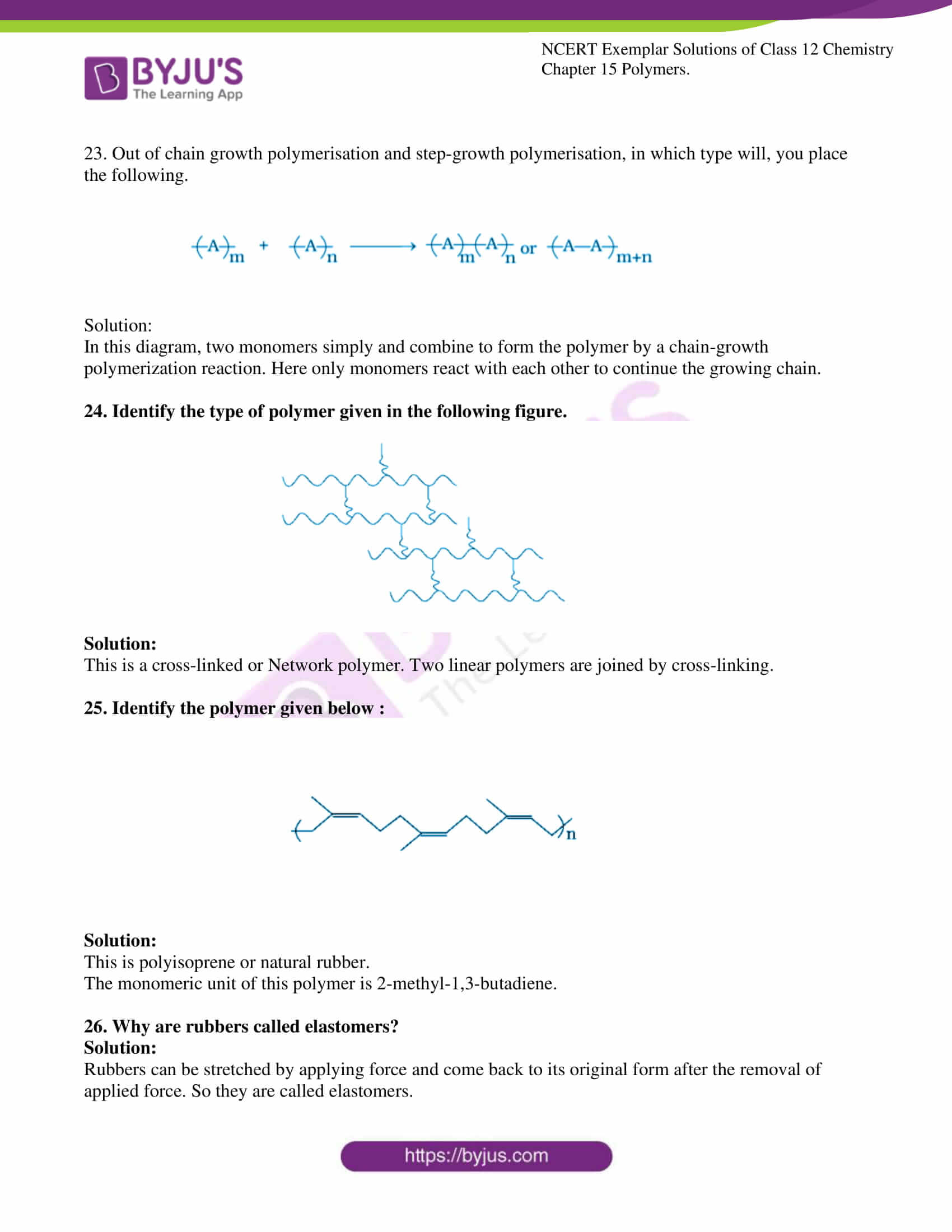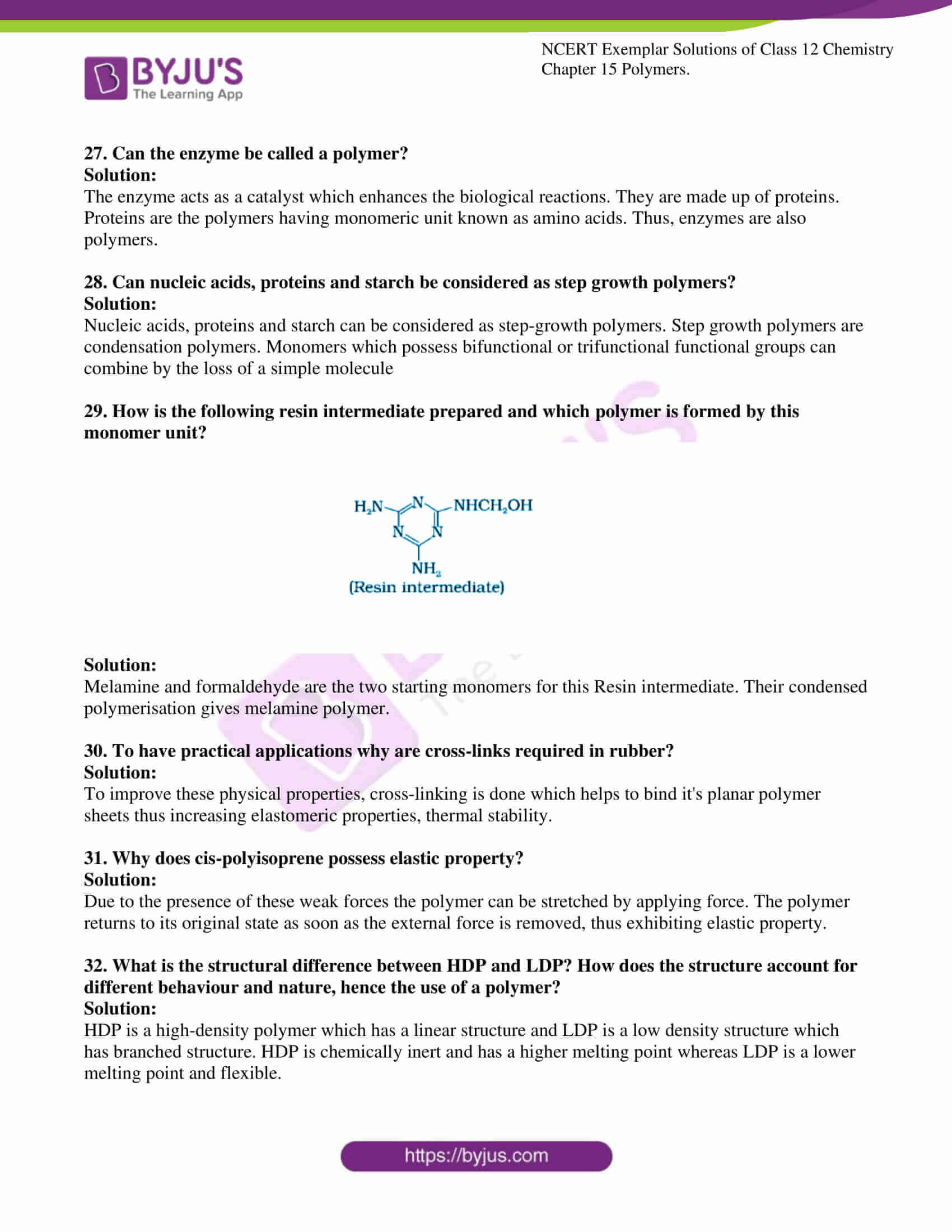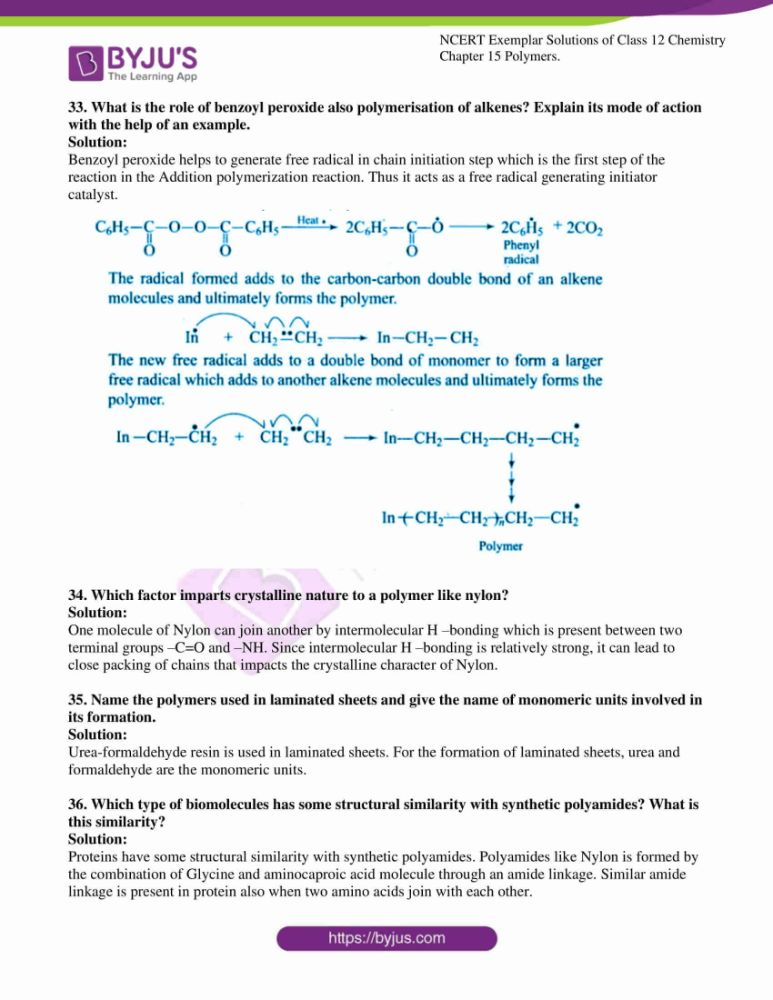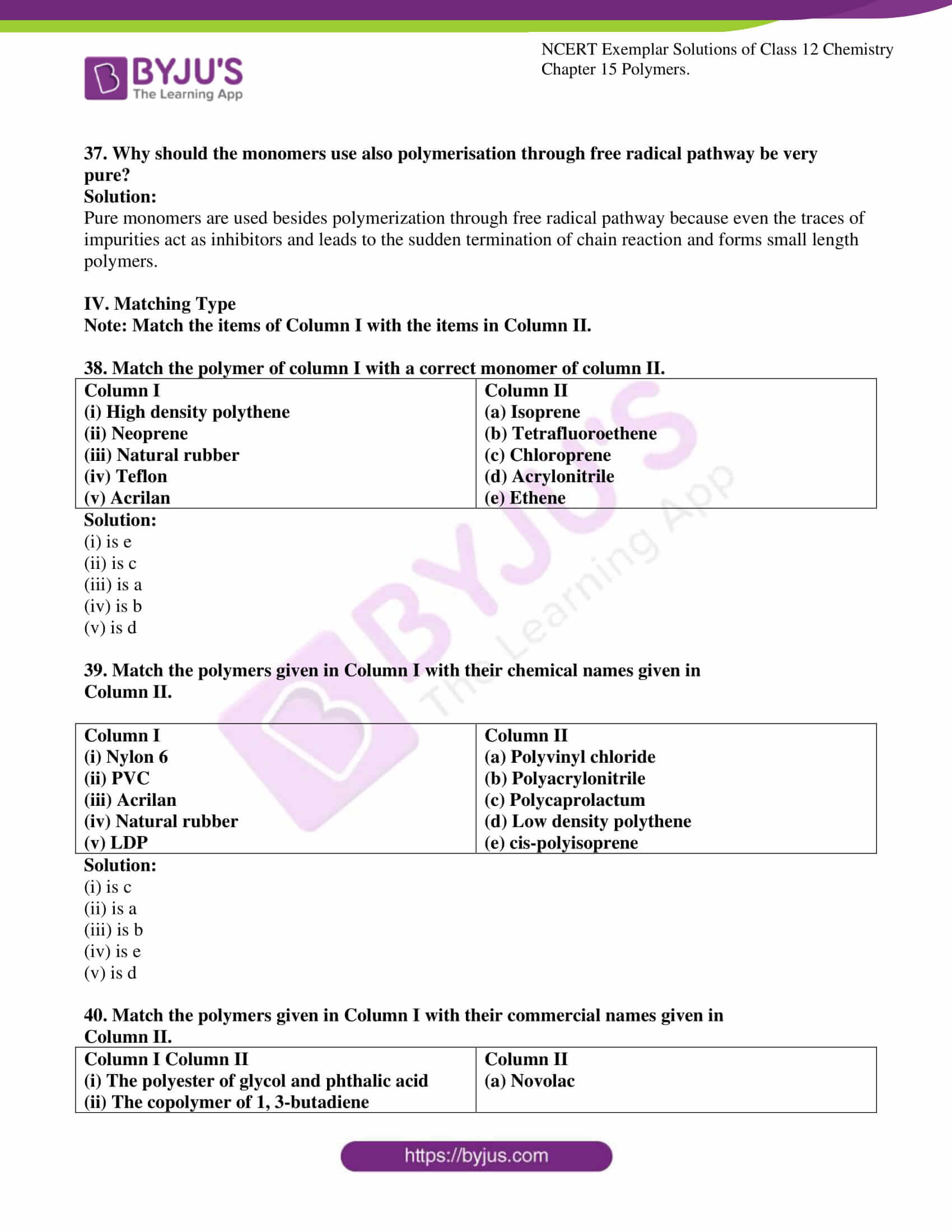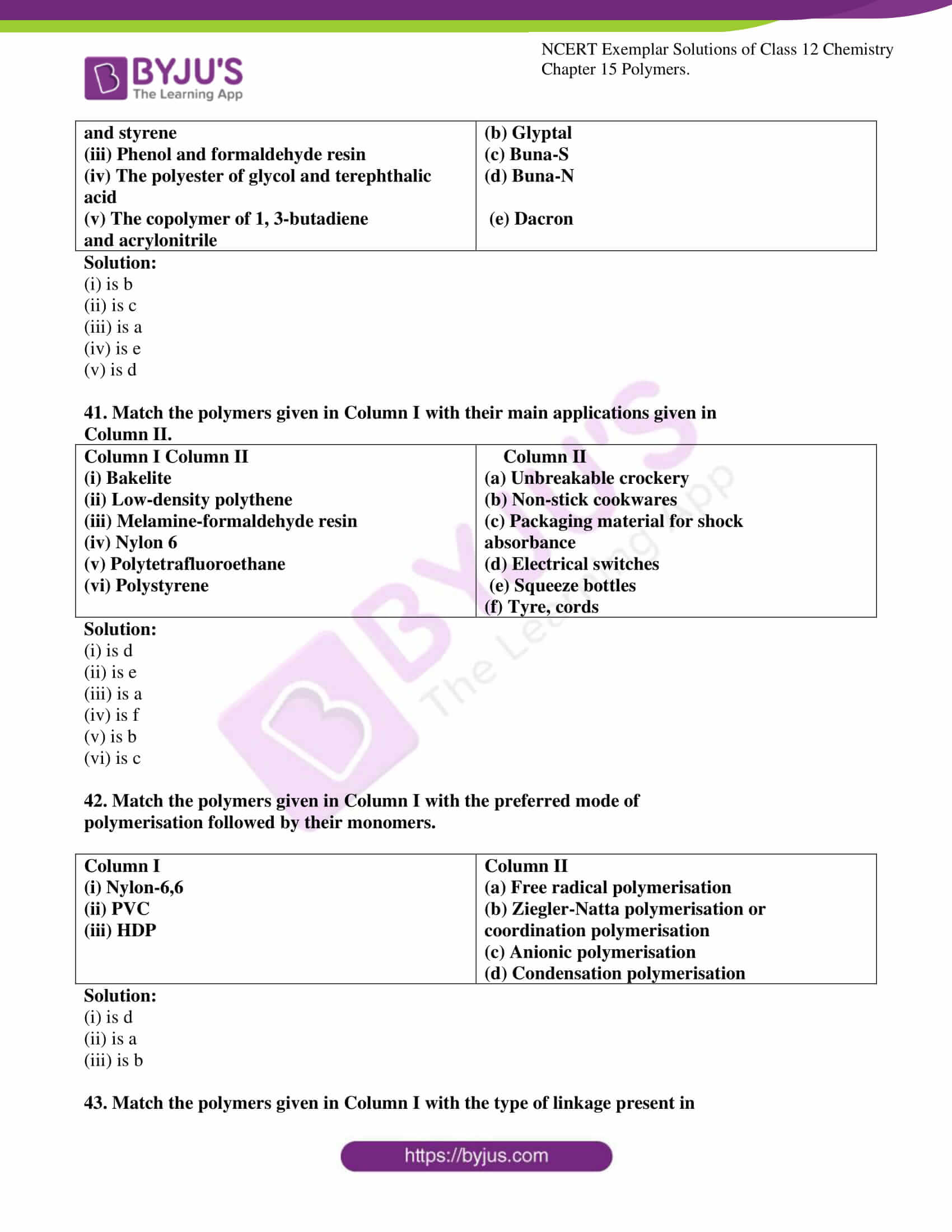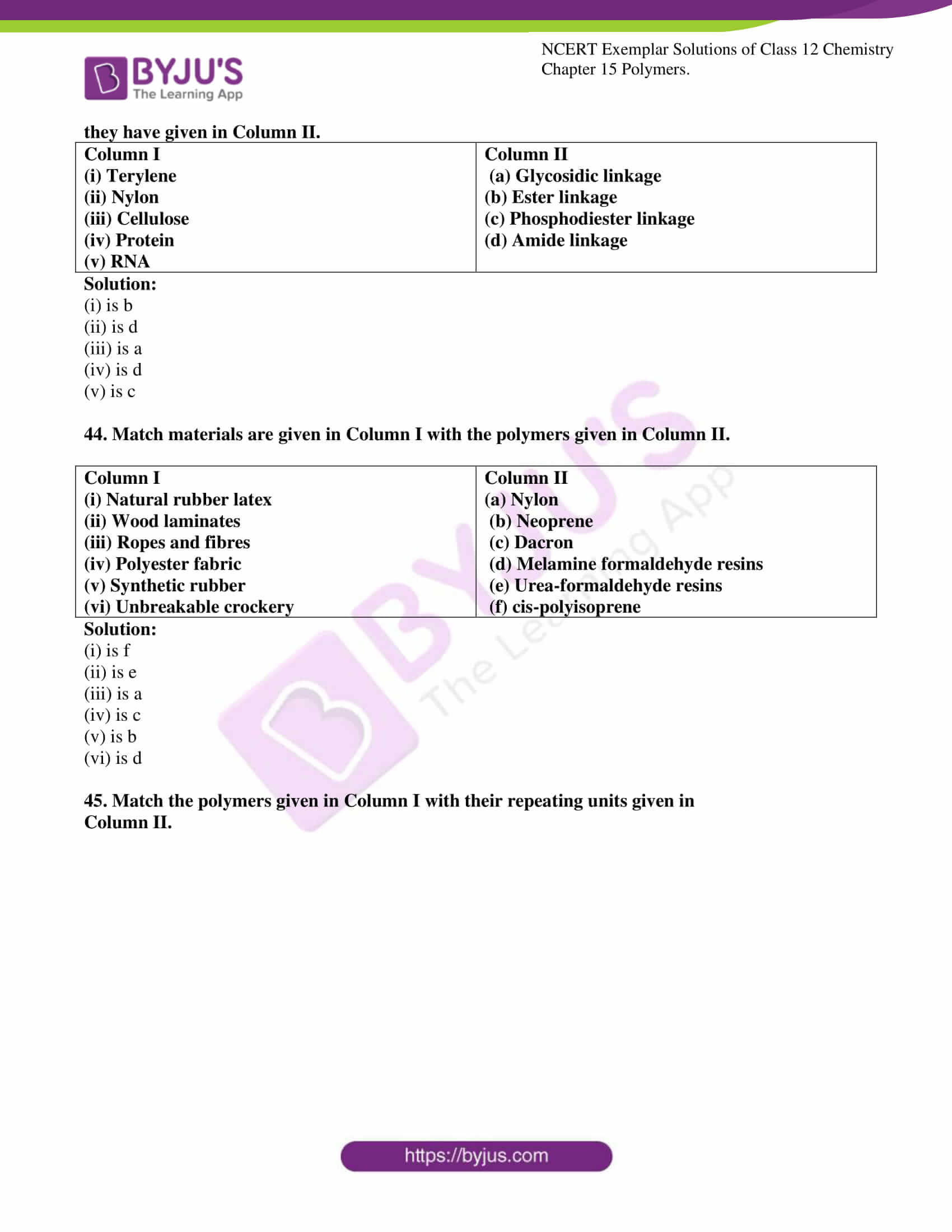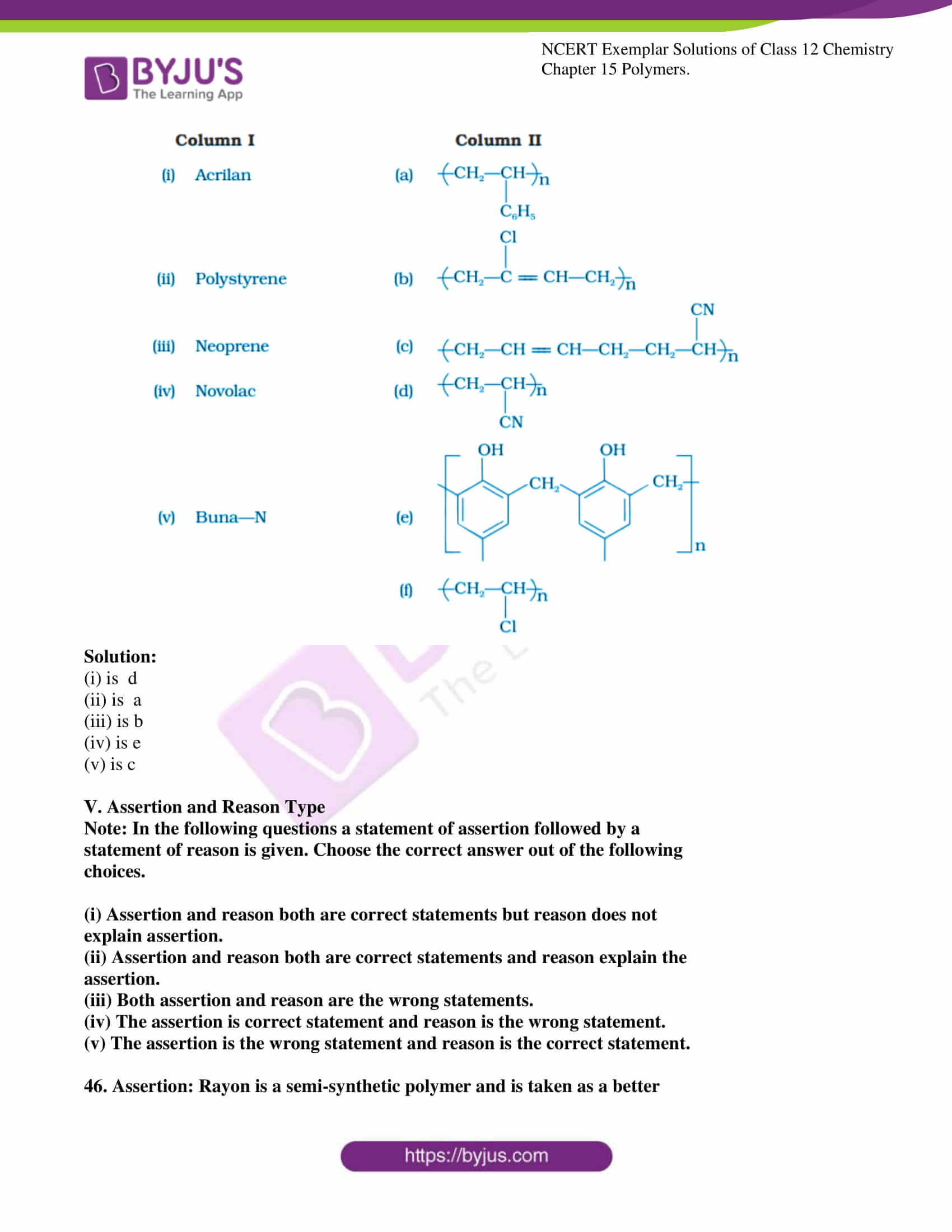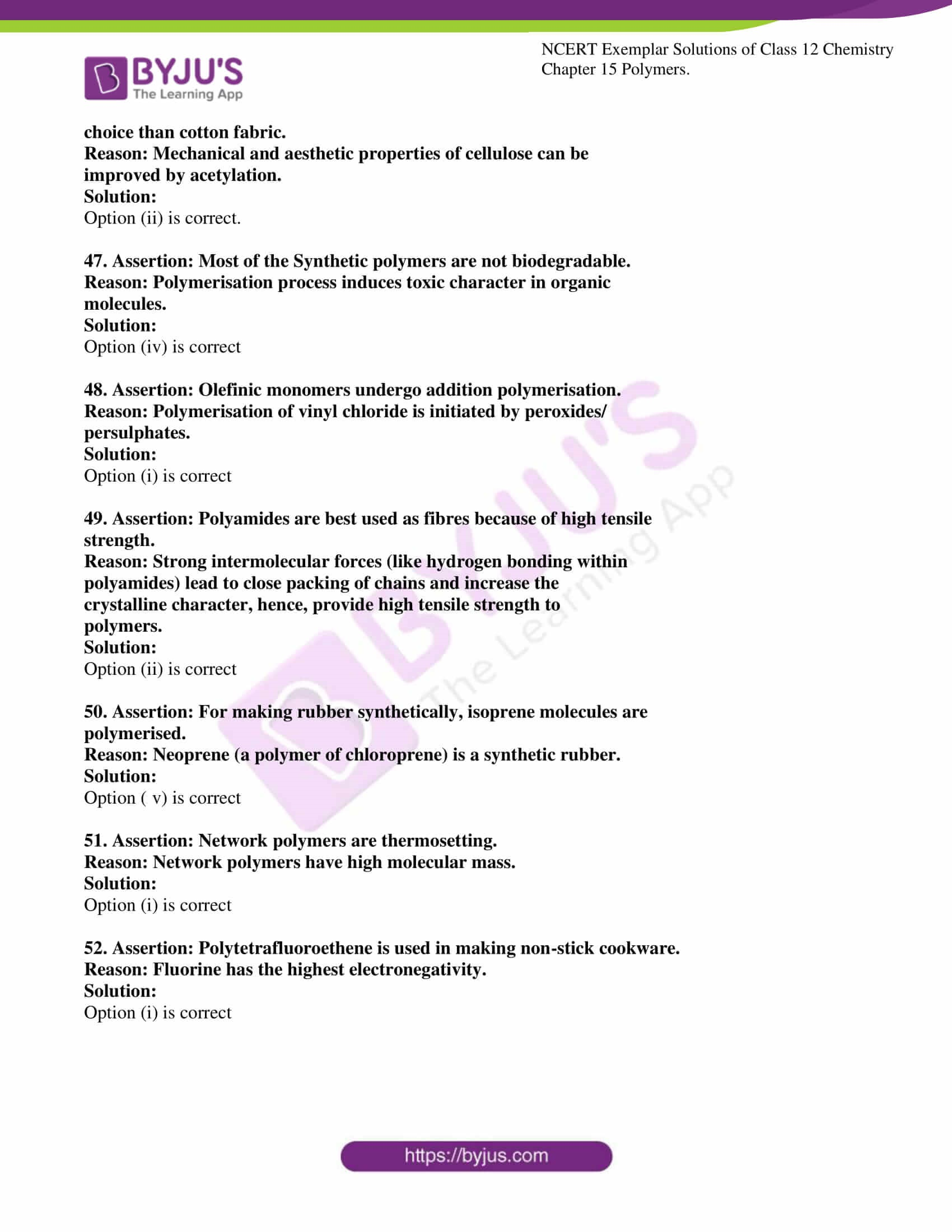### Access Solutions For NCERT Exemplar Class 12 Chemistry Chapter 15

I. Multiple Choice Questions (Type-I)

1. Which of the following polymers of glucose is stored by animals?

(i) Cellulose

(ii) Amylose

(iii) Amylopectin

(iv) Glycogen

Solution:

2. Which of the following is not a semisynthetic polymer?

(i) cis-polyisoprene

(ii) Cellulose nitrate

(iii) Cellulose acetate

(iv) Vulcanised rubber

Solution:

3. The commercial name of polyacrylonitrile is ______________.

(i) Dacron

(ii) Orlon (Acrilan)

(iii) PVC

(iv) Bakelite

Solution:

4. Which of the following polymer is biodegradable?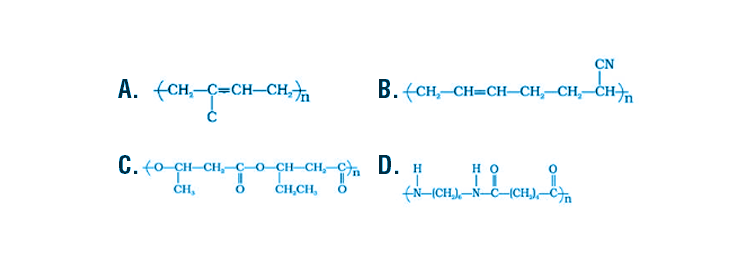Solution:

5. In which of the following polymers ethylene glycol is one of the monomer

units?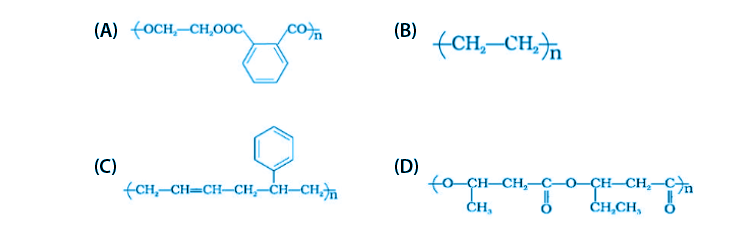Solution:

6. Which of the following statements is not true about low-density polythene?

(i) Tough

(ii) Hard

(iii) Poor conductor of electricity

(iv) Highly branched structure

Solution:

7.is a polymer having monomer units ____________.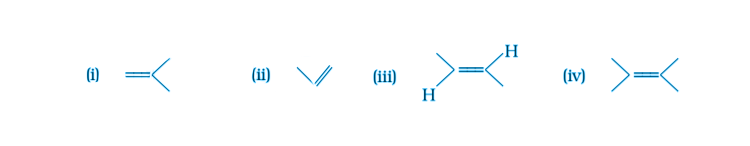Solution:

8. Which of the following polymer can be formed by using the following monomer

unit?Solution:

II. Multiple Choice Questions (Type-II)

Note: In the following questions two or more options may be correct.

9. Which of the following polymers, need at least one diene monomer for their

preparation?

(i) Dacron

(ii) Buna-S

(iii) Neoprene

(iv) Novolac

Solution:

Option (ii) and (iii) are the answers.

10. Which of the following are characteristics of thermosetting polymers?

(ii) Linear slightly branched long-chain molecules.

(iii) Become infusible on moulding so cannot be reused.

(iv) Soften on heating and harden on cooling, can be reused.

Solution:

Option (i) and (iii) are the answers.

11. Which of the following polymers are thermoplastic?

(i) Teflon

(ii) Natural rubber

(iii) Neoprene

(iv) Polystyrene

Solution:

Option (i) and (iv) are the answers.

12. Which of the following polymers are used as fibre?

(i) Polytetrafluoroethane

(ii) Polychloroprene

(iii) Nylon

(iv) Terylene

Solution:

Option (iii) and (iv) are the answers.

13. Which of the following are addition polymers?

(i) Nylon

(ii) Melamine formaldehyde resin

(iii) Orlon

(iv) Polystyrene

Solution:

Option (iii) and (iv) are the answers.

14. Which of the following polymers are condensation polymers?

(i) Bakelite

(ii) Teflon

(iii) Butyl rubber

(iv) Melamine formaldehyde resin

Solution:

Option (i) and (iv) are the answers.

15. Which of the following monomers form biodegradable polymers?

(i) 3-hydroxybutyric acid + 3-hydroxypentanoic acid

(ii) Glycine + aminocaproic acid

(iii) Ethylene glycol + phthalic acid

(iv) Caprolactam

Solution:

Option (i) and (ii) are the answers.

16. Which of the following is an example of a synthetic rubber?

(i) Polychloroprene

(ii) Polyacrylonitrile

(iii) Buna-N

(iv) cis-polyisoprene

Solution:

Option (i) and (iii) are the answers.

17. Which of the following polymers can have strong intermolecular forces?

(i) Nylon

(ii) Polystyrene

(iii) Rubber

(iv) Polyesters

Solution:

Option (i) and (iv) are the answers.

18. Which of the following polymers have vinylic monomer units?

(i) Acrilan

(ii) Polystyrene

(iii) Nylon

(iv) Teflon

Solution:

Option (i), (ii) and (iv) are the answers.

19. Vulcanisation makes rubber ______________.

(i) more elastic

(ii) soluble in inorganic solvent

(iii) crystalline

(iv) more stiff

Solution:

Option (i) and (iv) are the answers.

20. A natural linear polymer of 2-methyl-1, 3-butadiene becomes hard on

treatment with sulphur between 373 to 415 K and —S—S— bonds are formed

between chains. Write the structure of the product of this treatment?

Solution:This process is known as vulcanization of rubber. Sulphur here acts as a crosslinking agent and the rubber gets stiffened during this process having improved physical properties than natural rubber.

21. Identify the type of polymer.

—A—A—A—A—A—A—

Solution:

Here the monomer is –A-. Since the same or identical monomer is being repeated to form this above polymer, so this is a homopolymer. (‘Homo’ means same or identical)

22. Identify the type of polymer.

—A—B—B—A—A—A—B—A—

Solution:

Here the monomers A’ and ‘B’ are being joined in a random manner in the same polymer chain. So this is an example of a copolymer. A Copolymer is formed usually when the mixture of two or more unsaturated monomers react.

23. Out of chain growth polymerisation and step-growth polymerisation, in which type will, you place the following.Solution:

In this diagram, two monomers simply and combine to form the polymer by a chain-growth polymerization reaction. Here only monomers react with each other to continue the growing chain.

24. Identify the type of polymer given in the following figure.Solution:

This is a cross-linked or Network polymer. Two linear polymers are joined by cross-linking.

25. Identify the polymer given below :Solution:

This is polyisoprene or natural rubber.

The monomeric unit of this polymer is 2-methyl-1,3-butadiene.

26. Why are rubbers called elastomers?

Solution:

Rubbers can be stretched by applying force and come back to its original form after the removal of applied force. So they are called elastomers.

27. Can the enzyme be called a polymer?

Solution:

The enzyme acts as a catalyst which enhances the biological reactions. They are made up of proteins. Proteins are the polymers having monomeric unit known as amino acids. Thus, enzymes are also polymers.

28. Can nucleic acids, proteins and starch be considered as step growth polymers?

Solution:

Nucleic acids, proteins and starch can be considered as step-growth polymers. Step growth polymers are condensation polymers. Monomers which possess bifunctional or trifunctional functional groups can combine by the loss of a simple molecule

29. How is the following resin intermediate prepared and which polymer is formed by this monomer unit?Solution:

Melamine and formaldehyde are the two starting monomers for this Resin intermediate. Their condensed polymerisation gives melamine polymer.

30. To have practical applications why are cross-links required in rubber?

Solution:

To improve these physical properties, cross-linking is done which helps to bind it’s planar polymer sheets thus increasing elastomeric properties, thermal stability.

31. Why does cis-polyisoprene possess elastic property?

Solution:

Due to the presence of these weak forces the polymer can be stretched by applying force. The polymer returns to its original state as soon as the external force is removed, thus exhibiting elastic property.

32. What is the structural difference between HDP and LDP? How does the structure account for different behaviour and nature, hence the use of a polymer?

Solution:

HDP is a high-density polymer which has a linear structure and LDP is a low density structure which has branched structure. HDP is chemically inert and has a higher melting point whereas LDP is a lower melting point and flexible.

33. What is the role of benzoyl peroxide also polymerisation of alkenes? Explain its mode of action with the help of an example.

Solution:

Benzoyl peroxide helps to generate free radical in chain initiation step which is the first step of the reaction in the Addition polymerization reaction. Thus it acts as a free radical generating initiator catalyst.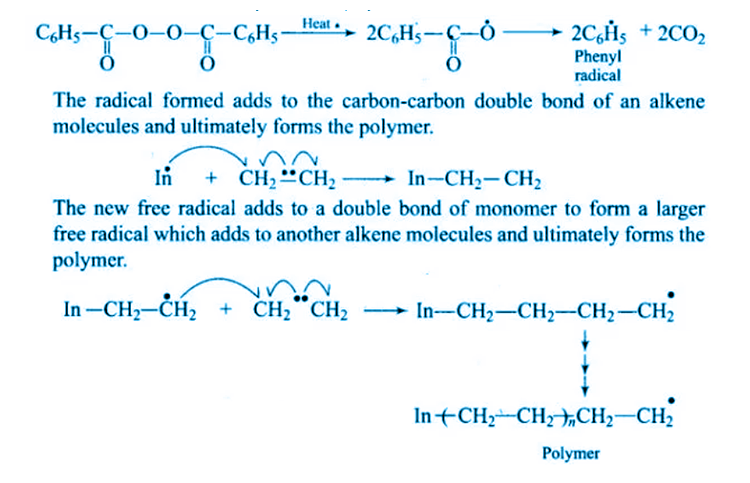34. Which factor imparts crystalline nature to a polymer like nylon?

Solution:

One molecule of Nylon can join another by intermolecular H –bonding which is present between two terminal groups –C=O and –NH. Since intermolecular H –bonding is relatively strong, it can lead to close packing of chains that impacts the crystalline character of Nylon.

35. Name the polymers used in laminated sheets and give the name of monomeric units involved in its formation.

Solution:

Urea-formaldehyde resin is used in laminated sheets. For the formation of laminated sheets, urea and formaldehyde are the monomeric units.

36. Which type of biomolecules has some structural similarity with synthetic polyamides? What is this similarity?

Solution:

Proteins have some structural similarity with synthetic polyamides. Polyamides like Nylon is formed by the combination of Glycine and aminocaproic acid molecule through an amide linkage. Similar amide linkage is present in protein also when two amino acids join with each other.

37. Why should the monomers use also polymerisation through free radical pathway be very pure?

Solution:

Pure monomers are used besides polymerization through free radical pathway because even the traces of impurities act as inhibitors and leads to the sudden termination of chain reaction and forms small length polymers.

IV. Matching Type

Note: Match the items of Column I with the items in Column II.

38. Match the polymer of column I with a correct monomer of column II.

 Column I (i) High density polythene (ii) Neoprene (iii) Natural rubber (iv) Teflon (v) Acrilan Column II (a) Isoprene (b) Tetrafluoroethene (c) Chloroprene (d) Acrylonitrile (e) Ethene

Solution:

(i) is e

(ii) is c

(iii) is a

(iv) is b

(v) is d

39. Match the polymers given in Column I with their chemical names given in

Column II.

 Column I (i) Nylon 6 (ii) PVC (iii) Acrilan (iv) Natural rubber (v) LDP Column II (a) Polyvinyl chloride (b) Polyacrylonitrile (c) Polycaprolactum (d) Low density polythene (e) cis-polyisoprene

Solution:

(i) is c

(ii) is a

(iii) is b

(iv) is e

(v) is d

40. Match the polymers given in Column I with their commercial names given in

Column II.

 Column I Column II (i) The polyester of glycol and phthalic acid (ii) The copolymer of 1, 3-butadiene and styrene (iii) Phenol and formaldehyde resin (iv) The polyester of glycol and terephthalic acid (v) The copolymer of 1, 3-butadiene and acrylonitrile Column II (a) Novolac (b) Glyptal (c) Buna-S (d) Buna-N (e) Dacron

Solution:

(i) is b

(ii) is c

(iii) is a

(iv) is e

(v) is d

41. Match the polymers given in Column I with their main applications given in

Column II.

 Column I Column II (i) Bakelite (ii) Low-density polythene (iii) Melamine-formaldehyde resin (iv) Nylon 6 (v) Polytetrafluoroethane (vi) Polystyrene Column II (a) Unbreakable crockery (b) Non-stick cookwares (c) Packaging material for shock absorbance (d) Electrical switches (e) Squeeze bottles (f) Tyre, cords

Solution:

(i) is d

(ii) is e

(iii) is a

(iv) is f

(v) is b

(vi) is c

42. Match the polymers given in Column I with the preferred mode of

polymerisation followed by their monomers.

 Column I (i) Nylon-6,6 (ii) PVC (iii) HDP Column II (a) Free radical polymerisation (b) Ziegler-Natta polymerisation or coordination polymerisation (c) Anionic polymerisation (d) Condensation polymerisation

Solution:

(i) is d

(ii) is a

(iii) is b

43. Match the polymers given in Column I with the type of linkage present in

they have given in Column II.

 Column I (i) Terylene (ii) Nylon (iii) Cellulose (iv) Protein (v) RNA Column II (a) Glycosidic linkage (b) Ester linkage (c) Phosphodiester linkage (d) Amide linkage

Solution:

(i) is b

(ii) is d

(iii) is a

(iv) is d

(v) is c

44. Match materials are given in Column I with the polymers given in Column II.

 Column I (i) Natural rubber latex (ii) Wood laminates (iii) Ropes and fibres (iv) Polyester fabric (v) Synthetic rubber (vi) Unbreakable crockery Column II (a) Nylon (b) Neoprene (c) Dacron (d) Melamine formaldehyde resins (e) Urea-formaldehyde resins (f) cis-polyisoprene

Solution:

(i) is f

(ii) is e

(iii) is a

(iv) is c

(v) is b

(vi) is d

45. Match the polymers given in Column I with their repeating units given in

Column II.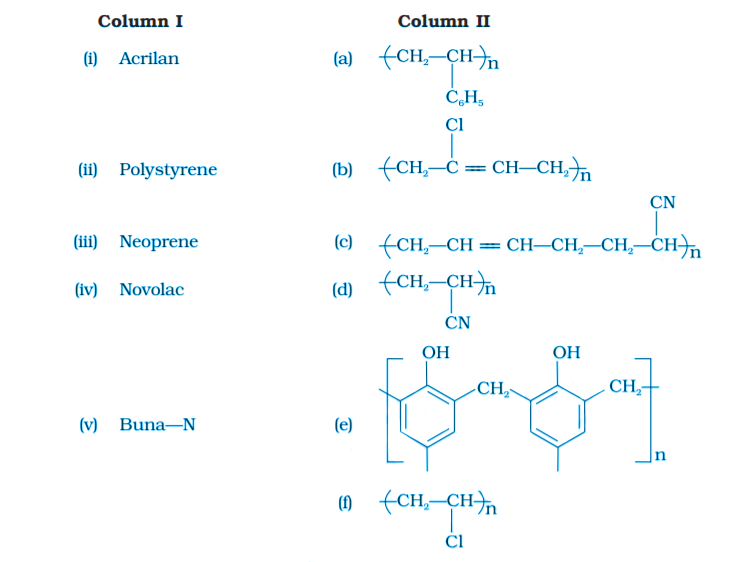Solution:

(i) is d

(ii) is a

(iii) is b

(iv) is e

(v) is c

V. Assertion and Reason Type

Note: In the following questions a statement of assertion followed by a

statement of reason is given. Choose the correct answer out of the following

choices.

(i) Assertion and reason both are correct statements but reason does not

explain the assertion.

(ii) Assertion and reason both are correct statements and reason explain the

assertion.

(iii) Both assertion and reason are the wrong statements.

(iv) The assertion is correct statement and reason is the wrong statement.

(v) The assertion is the wrong statement and reason is the correct statement.

46. Assertion: Rayon is a semi-synthetic polymer and is taken as a better

choice than cotton fabric.

Reason: Mechanical and aesthetic properties of cellulose can be

improved by acetylation.

Solution:

Option (ii) is correct.

47. Assertion: Most of the Synthetic polymers are not biodegradable.

Reason: Polymerisation process induces toxic character in organic

molecules.

Solution:

Option (iv) is correct

48. Assertion: Olefinic monomers undergo addition polymerisation.

Reason: Polymerisation of vinyl chloride is initiated by peroxides/

persulphates.

Solution:

Option (i) is correct

49. Assertion: Polyamides are best used as fibres because of high tensile

strength.

Reason: Strong intermolecular forces (like hydrogen bonding within

polyamides) lead to close packing of chains and increase the

crystalline character, hence, provide high tensile strength to

polymers.

Solution:

Option (ii) is correct

50. Assertion: For making rubber synthetically, isoprene molecules are

polymerised.

Reason: Neoprene (a polymer of chloroprene) is a synthetic rubber.

Solution:

Option (v) is correct

51. Assertion: Network polymers are thermosetting.

Reason: Network polymers have high molecular mass.

Solution:

Option (i) is correct

52. Assertion: Polytetrafluoroethene is used in making non-stick cookware.

Reason: Fluorine has the highest electronegativity.Solution:

Option (i) is correct

### Class 12 Chemistry NCERT Exemplar Polymers

Polymers are one of the most useful inventions of all times. We use substances made of polymers every day. Like the handle of a rice cooker, the comb we use to comb our hair. In this chapter, students will learn about different types of polymers and their uses. To practice questions from this chapter students are advised to solve NCERT exemplar Class 12 Chemistry Chapter 15 Polymers.

#### Important topics of Class 12 Chemistry Chapter 15 Polymers Monomer

1. Classification of Polymers
2. Types of Polymerisation Reactions
3. Molecular Mass of Polymers